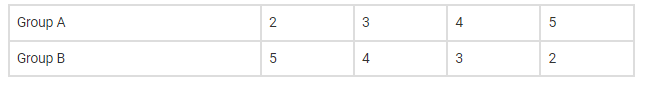# A candidate is required to answer 7 questionsQuestion:

A candidate is required to answer 7 questions out of 12 questions, which are divided into two groups, each containing 6 questions. He is not permitted to attempt more than 5 questions from either group. Find the number of different ways of doing questions.

Solution:

We know that,

nCr

$=\frac{n !}{r !(n-r) !}$

No of questions in group A=6

No of questions in group B=6

According to the question,

The different ways in which the questions can be attempted are,Hence, the number of different ways of doing questions,

= (6C2x6C5)+(6C3x6C4)+(6C4x6C3)+(6C5x6C2)

= (15×6)+(20×15)+(15×20)+(6×15)

=780